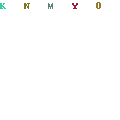How To Calculate ConcreteRemember: 1 Cubic Yard of Concrete = 3′ x 3′ x 3′ = 27 cubic feet = 46,656 cubic inches This is an exercise aimed at a real world example of how to calculate concrete yardage needed to order for your slab placement. The given’s for this exercise in how to calculate concrete are as … [Read more…]